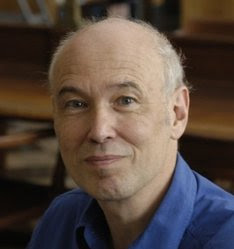### Pierre Deligne and the Weil conjecturesPierre Deligne, a belgian mathematician, wins the Abel Prize 2013
for seminal contributions to algebraic geometry and for their transformative impact on number theory, representation theory, and related fields
One of the most famous contribution by Deligne was the proof of one of the three Weil conjectures. These conjectures was stated by Andre Weil, the mathematician who proofed the Fermat's last theorem, in 1949 (Numbers of solutions of equations in finite fields) in order to solve the following problem:
how to count the number of solutions to systems of polynomial equations over finite fields(1)
In particular
Weil conjectured that such zeta-functions should be rational functions, should satisfy a form of functional equation, and should have their zeroes in restricted places. The last two parts were quite consciously modeled on the Riemann zeta function and Riemann hypothesis. The rationality was proved by Dwork (1960), the functional equation by Grothendieck (1965), and the analogue of the Riemann hypothesis was proved by Deligne (1974)
The conjectures stated(1):
1. $\zeta_V (t)$ is a rational function of $t$;
2. more explicitly, if $n = \dim V$, we can write $\zeta_V (t) = \frac{P_1 (t) P_3 (t) \cdots P_{2n−1} (t)}{P_0 (t) P_2 (t) \cdots P_{2n} (t)}$ where each root of each $P_i (t)$ is a complex number of norm $q^{−i/2}$;
3. the roots of $P_i (t)$ are interchanged with the roots of $P_{2n−i} (t)$ under the substitution $t \rightarrow 1/q^n t$;
4. if $V$ is the reduction modulo $p$ of a variety $\tilde{V}$ defined over a subfield of $\mathbb{C}$, then $b_i := \deg P_i (t)$ is the $i$th topological Betti number of $\tilde{V}$ using the usual topology.
where $V$ is a higher-dimensional variety over a finite fields.
Deligne's powerful concepts, ideas, results and methods continue to influence the development of algebraic geometry, as well as mathematics as a whole

Deligne's proof of the Weil conjecture connected to the Riemann hypotesis are published in the two papers La conjecture de Weil: I and La conjecture de Weil: II
(1) Brian Osserman. The Weil conjectures (pdf)
(2) Wikipedia: Weil conjectures